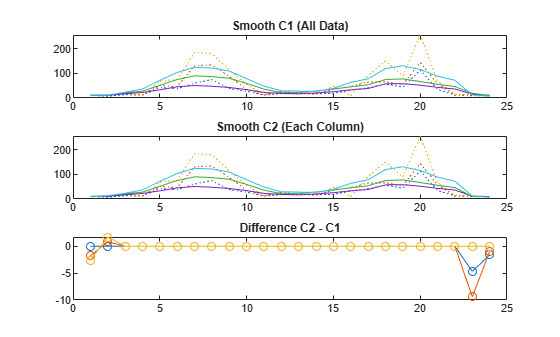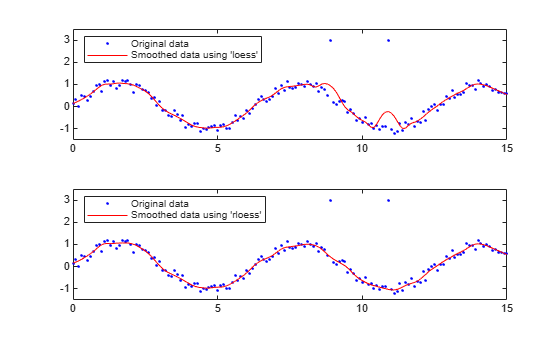# smooth

Smooth response data

## Syntax

``yy = smooth(y)``
``yy = smooth(y,span)``
``yy = smooth(y,method)``
``yy = smooth(y,span,method)``
``yy = smooth(y,'sgolay',degree)``
``yy = smooth(y,span,'sgolay',degree)``
``yy = smooth(x,y,___)``
``gpuarrayYY = smooth(gpuarrayY,___)``
``gpuarrayYY = smooth(gpuarrayX,gpuarrayY,___)``

## Description

example

````yy = smooth(y)` smooths the response data in column vector `y` using a moving average filter.The first few elements of `yy` follow.yy(1) = y(1) yy(2) = (y(1) + y(2) + y(3))/3 yy(3) = (y(1) + y(2) + y(3) + y(4) + y(5))/5 yy(4) = (y(2) + y(3) + y(4) + y(5) + y(6))/5 ...Because of the way `smooth` handles endpoints, the result differs from the result returned by the `filter` function.```
````yy = smooth(y,span)` sets the span of the moving average to `span`.```
````yy = smooth(y,method)` smooths the data in `y` using the method specified by `method` and the default `span`.```

example

````yy = smooth(y,span,method)` sets the span of `method` to `span`.```
````yy = smooth(y,'sgolay',degree)` uses the Savitzky-Golay method with the polynomial degree specified by `degree`.```
````yy = smooth(y,span,'sgolay',degree)` uses the number of data points specified by `span` in the Savitzky-Golay calculation. `span` must be odd and `degree` must be less than `span`.```

example

````yy = smooth(x,y,___)` specifies values for the independent variable `x`. You can use this syntax with any of the arguments in the previous syntaxes. ```
````gpuarrayYY = smooth(gpuarrayY,___)` performs the operation on a GPU using `gpuArray` data. You can use `gpuArray` response data with all previous syntaxes. This syntax requires Parallel Computing Toolbox™. ```
````gpuarrayYY = smooth(gpuarrayX,gpuarrayY,___)` performs the operation on a GPU using `gpuArray` input data. This syntax requires Parallel Computing Toolbox. NoteUsing `gpuArray` `x` and `y` inputs with the `smooth` function is only recommended if you use the default method, `'moving'`. Using GPU data with other methods does not offer any performance advantage. ```

## Examples

collapse all

Smooth data by linear index and by each column separately, using a moving average filter. Plot and compare the results.

Load the data in `count.dat`. The 24-by-3 array `count` contains traffic counts at three intersections for each hour of the day.

`load count.dat`

Suppose that the data are from a single intersection over three consecutive days. Smoothing all the data together would then indicate the overall cycle of traffic flow through the intersection. Use a moving average filter with a 5-hour span to smooth all the data simultaneously (by linear index).

```c = smooth(count(:)); C1 = reshape(c,24,3);```

However, the data are in fact from three different intersections. Thus, smoothing columnwise gives a more meaningful picture of the traffic through each intersection in a day. Use the same moving average filter to smooth each column of the data separately.

```C2 = zeros(24,3); for I = 1:3 C2(:,I) = smooth(count(:,I)); end```

Plot the original data and the data smoothed by linear index and by each column separately. Then, plot the difference between the two smoothed data sets. The two methods give different results near the endpoints.

```subplot(3,1,1) plot(count,':'); hold on plot(C1,'-'); title('Smooth C1 (All Data)') subplot(3,1,2) plot(count,':'); hold on plot(C2,'-'); title('Smooth C2 (Each Column)') subplot(3,1,3) plot(C2 - C1,'o-') title('Difference C2 - C1')```Plot and compare the results of data smoothed using the `loess` and `rloess` methods. Then determine which method is less sensitive to outliers.

Create noisy data with two outliers.

```x = (0:0.1:15)'; y = sin(x) + 0.5*(rand(size(x))-0.5); y([90,110]) = 3;```

Smooth the data with the `loess` and `rloess` methods. Use a span of 10% of the total number of data points.

```yy1 = smooth(x,y,0.1,'loess'); yy2 = smooth(x,y,0.1,'rloess');```

Plot the original and smoothed data. The outliers have less influence with the robust method `rloess`.

```subplot(2,1,1) plot(x,y,'b.',x,yy1,'r-') set(gca,'YLim',[-1.5 3.5]) legend('Original data','Smoothed data using ''loess''',... 'Location','NW') subplot(2,1,2) plot(x,y,'b.',x,yy2,'r-') set(gca,'YLim',[-1.5 3.5]) legend('Original data','Smoothed data using ''rloess''',... 'Location','NW')```## Input Arguments

collapse all

Data to smooth, specified as a column vector.

If your data contains `NaN`s and you do not specify `x`, your data is treated as nonuniform and the smoothing method `'lowess'` is used.

Data Types: `single` | `double`

Number of data points for calculating the smoothed value, specified as an integer or as a scalar value in the range (0,1) denoting a fraction of the total number of data points.

If you use the moving average or Savitzky-Golay methods, the number of data points for calculating the smoothed value must be an odd integer. If you specify `span` as an even number or as a fraction that results in an even number of data points, `span` is automatically reduced by 1.

Example: `7`; `0.6`

Smoothing method to smooth the response data, specified as one of the following methods.

Method

Description

`'moving'` (default)

Moving average. A lowpass filter with filter coefficients equal to the reciprocal of the span.

`'lowess'`

Local regression using weighted linear least squares and a 1st degree polynomial model.

`'loess'`

Local regression using weighted linear least squares and a 2nd degree polynomial model.

`'sgolay'`

Savitzky-Golay filter. A generalized moving average with filter coefficients determined by an unweighted linear least-squares regression and a polynomial model of specified degree (default is 2). The method can accept nonuniform predictor data.

`'rlowess'`

A robust version of `'lowess'` that assigns lower weight to outliers in the regression. The method assigns zero weight to data outside six mean absolute deviations.

`'rloess'`

A robust version of `'loess'` that assigns lower weight to outliers in the regression. The method assigns zero weight to data outside six mean absolute deviations.

Data Types: `char` | `string`

Polynomial degree for the model used in the Savitzky-Golay method, specified as a scalar value. `degree` must be less than `span`.

Example: `3`

Independent variable for the response data `y`, specified as a column vector. If you do not provide `x`, methods that require `x` assume `x = 1:length(y)`. Specify `x` data when `y` is not sorted or uniformly spaced. If `x` is not uniform and you do not specify `method`, `lowess` is used. If you specify a smoothing method that requires `x` to be sorted, the function automatically sorts the `x` data.

Data Types: `single` | `double`

Data to smooth, specified as a `gpuArray` column vector.

Data Types: `single` | `double`

Input data for the response data `gpuarrayY`, specified as a `gpuArray` column vector. If you do not provide `gpuarrayX`, methods that require `gpuarrayX` assume `gpuarrayX = 1:length(y)`. Specify `gpuarrayX` data when the data are not uniformly spaced or sorted. If the `gpuarrayX` data is not uniform and you do not specify the smoothing method, `'lowess'` is used. If you specify a smoothing method that requires `gpuarrayX` to be sorted, the function automatically sorts the `gpuarrayX` data.

Data Types: `single` | `double`

## Output Arguments

collapse all

Smoothed data, returned as a column vector.

Smoothed data, returned as a `gpuArray` column vector.

## Tips

• For more options for smoothing data, including the moving median and Gaussian methods, see `smoothdata`.

• You can generate a smooth fit to your data using a smoothing spline. For more information, see `fit`.

## Version History

Introduced before R2006a